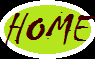## Sunday, April 14, 2013

### PL/SQL Functions Overview with Examples

Functions:
=======
A function is a named PL/SQL Block which is similar to a procedure. The major difference between a procedure and a function is, a function must always return a value, but a procedure may or may not return a value.

Functions are divided into two types
• System defined functions
• User defined functions
System defined functions: A function which is defined by the system automatically those functions can be called as system defined functions.

User defined functions: A function which is defined by user manually those functions can be called as user defined functions.

--> User defined functions are further divided into four types, depending on receiving and sending the values.
• A function which receives and returns the value
• A function which receives but doesn’t returns the value
• A function doesn’t receive but returns the value
• A function doesn’t receive and doesn’t return the value
Functions are used to estimate or calculate any value. In PL/SQL function contains two sections.
1) Declaration of the function
2) Body of the function

A declaration of the function starts with keyword called create and ends with return statement, whereas body of the function starts with a keyword called is and ends with end statement.

Syntax :
=====

Create [OR Replace] function function_name [parameters]
return return_datatype;
IS
Declaration_section
Begin
Execution_section
Return return_variable;
Exception
exception section
return return_variable;
End;

Example-1 :write a function input two numbers and find out its sum.

SQL>Create function add(a in number, b in number)
Return number
Is
Begin
c:=a+b;
return c;
End;
/
Function created

Execution :
=======
SQL> var x number

PL/SQL procedure successfully completed

SQL> print x

X
---------------------------------------------
15

Example-2 :write a function input any number and find the factorial.

SQL>Create or replace function fact(n number)
Return number
Is
i number;
f number:=1;
Begin
for i in 1..n loop
f:=f*i;
End loop;
return f;
End;
/
Function created

Execution :
=======

SQL> var x number
SQL> exec :x:=fact(5);
PL/SQL procedure successfully completed

SQL> print x

X
---------------------------------------------
120

we consider the following two tables i.e emp, dept to write SQL queries in the coming PL/SQL programs.

Double click on the image to view the full image with clarityExample-3 :write a function to find the maximum salary in employee table.

SQL>Create or replace function bigsal
Return number
Is
c number;
Begin
select max(sal) into c from emp;
Return c;
End;
/
Function created

Execution :
=======

SQL> var x number
SQL> exec :x:=bigsal()
PL/SQL Procedure Successfully Completed

SQL> print x

X
---------------------------------------------
5000

Example-4 :write a function to count the number of employees working in sales department.

SQL>Create or replace function countsales
Return number
Is
c number;
Begin
select count(*) into c from emp where deptno=(select deptno from dept where dname=’SALES’);
Return c;
End;
/
Function created

Execution :
=======

SQL> var x number
SQL> exec :x:=countsales()
PL/SQL Procedure Successfully Completed

SQL> print x

X
---------------------------------------------
6

Example-5 :write a function to display the minimum salary employee name working in accounts department.

SQL>Create or replace function ename_minsal
Return varchar2
Is
c varchar2(20);
Begin
select ename into c from emp where sal=(select min(sal) from emp
where deptno=(select deptno from dept where dname=’ACCOUNTING’));
return c;
End;
/
Function created

Execution :
=======

SQL> var y varchar2(20)
SQL> exec :y:=ename_minsal()
PL/SQL Procedure Successfully Completed

SQL> print y

Y
---------------------------------------------
MILLER

Example-6 :write a function to display the hire date of an employee who are having the maximum commission.

SQL>Create or replace function hiredatefn
Return date
Is
c date;
Begin
select hiredate into c from emp where comm=(select max(comm) from emp);
Return c;
End;

SQL> var y varchar2(15); //should not take variable as date use varchar, number..etc
SQL> exec :y:=hiredatefn()
PL/SQL function successfully completed

SQL> print y

Y
---------------------------------------------
28-SEP-81

Example-7 :write a function to count number of employees working in sales and accounts department.

SQL>Create or replace function count_emp
Return number
Is
c number;
Begin
select count(*) into c from emp
where deptno in(select deptno from dept where dnmae in(‘SALES’,’ACCOUNTING’));
Return c;
End;
/
Function created

Execution :
=======
SQL> var z number
SQL> exec :z:=count_emp()
PL/SQL Procedure Successfully Completed

SQL> print z

Z
---------------------------------------------
9

Example-8 :write a function to count number of employees whose salaries is greater than any employee salary working in 20th department.

SQL>Create or replace function count_emp
Return number
Is
c number;
Begin
Select count(*) into c from emp where sal> any(select max(sal) from emp where deptno=20);
Return c;
End;
/
Function created

Execution :
=======

SQL> var a number
SQL> exec :a:=count_emp()
PL/SQL Procedure Successfully Completed

SQL> print a

A
---------------------------------------------
1

Example-9 :write a function to find sum of the salaries in 20th department.

SQL>Create or replace function sumsal
Return number
Is
c number;
Begin
select sum(sal) from emp where deptno=20;
Return c;
End;
/
Function created

Execution :
=======
SQL> var a number
SQL> exec :a:=sumsal()
PL/SQL Procedure Successfully Completed

SQL> print a

A
---------------------------------------------
10875

--> Syntax to see the list of all sub programs

SQL> select * from user_source;

--> Syntax to see the source code of a particular program

SQL> select text from user_source where name=’<sub program name>’;

--> Syntax to drop the package

SQL> drop function <function_name>;